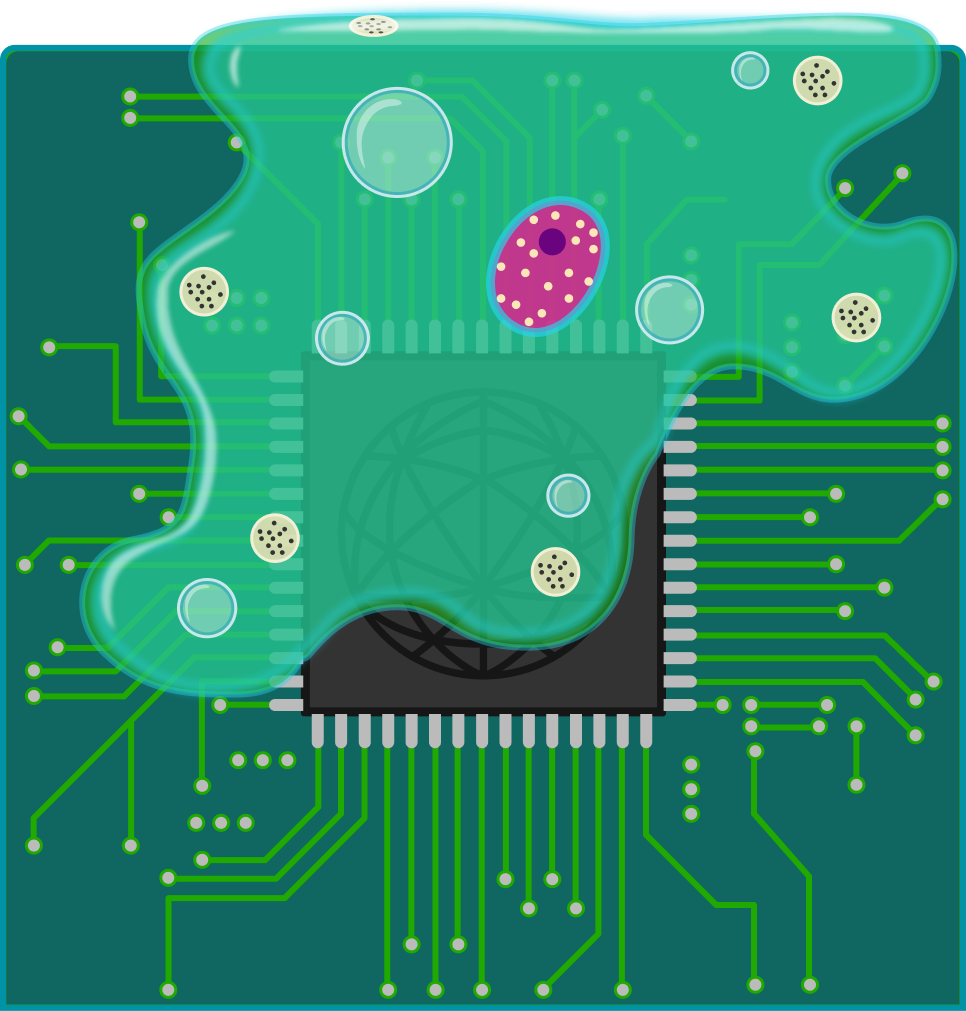# Exploding Genomes

The first explosion of a nuclear bomb was the Trinity test conducted in New Mexico in July 1945. The test was conducted in secret, and even after two nuclear bombs were dropped on Japan to end the war, most details about nuclear weapons, including their incredible energetic yields, remained a closely guarded secret. This didn't keep the people in charge from publishing a series of photos of the Trinity explosion, along with a size scale and time stamps on the cover of Life magazine.Perhaps they just wanted to show off, but based on these photographs a British physicist named G. I. Taylor was able to estimate the yield of the explosion, an estimate that was incredibly close to the secret value of the actual yield. His estimation didn't take any knowledge of nuclear physics or any details about the construction of the bomb. He just made a simple toy model of an expanding shock wave based on fluid mechanics and dimensional analysis.

At many points in this course, we won't dive into the details of the chemistry or biology of a problem, but we too will use simple toy models to gain useful insights.

## Exploding Genomes

### Biological Numeracy

# Exploding Genomes

We've already seen that genomes contain a great deal of information. If you scale the information density of a viral genome up to the volume of a memory card, it could back up the entire internet several times over. It's tricky business packing that much into the nucleus of a cell, or the capsid of a virus, some of which store DNA at an incredible $$50 \textrm{ atm}$$ of internal pressure.

In the early days of molecular biology, no one had any idea of how big genomes were. A great deal of insight into all kinds of biological structures was gained from looking at cells and viruses under electron microscopes. One of these insights was the dense packing of DNA: if cells or viruses weren't prepared correctly, they'd often explode while being observed on the microscope, leaving a blast radius of randomly strewn DNA.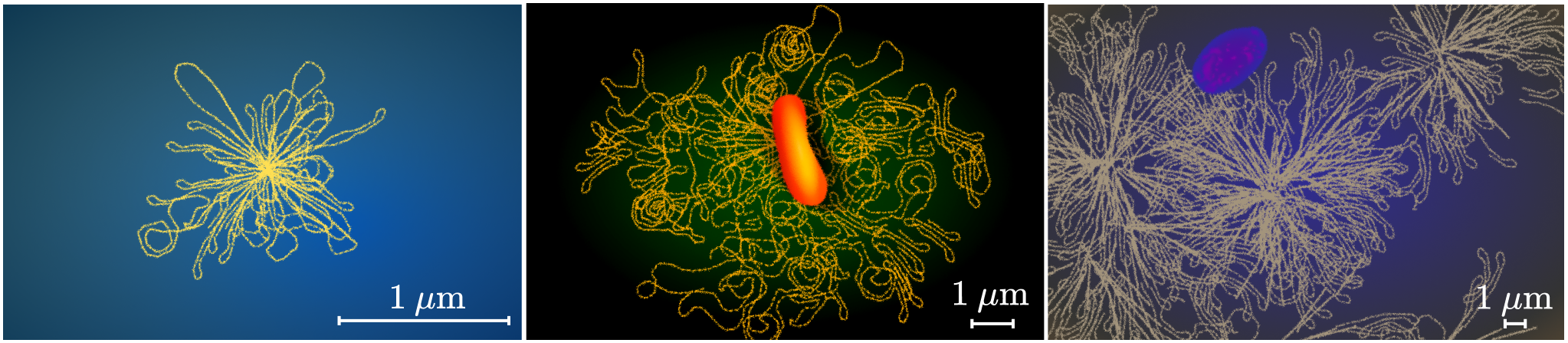In the spirit of G. I. Taylor, let's estimate the number of nucleotides and the amount of information contained in viral, bacterial, and human genomes based only on their blast radii. Along the way, we'll get to know the type of toy models that will come in handy many times in this course.

## Exploding Genomes

### Biological Numeracy

# Exploding Genomes

When it comes to biological structures, DNA is pretty much the simplest of the bunch. It's a long chain of four different molecules which encodes data. We'll explore the structure and function of DNA in detail in the next chapter, but for now, let's treat it like a chain. Our goal here is to figure out how many links are in the chains in the exploded genomes we just saw. Once we know how many links there are, it's straightforward to estimate the information content.

DNA chains come in two forms. Single-stranded DNA is a long, flexible molecule with three bendable joints every nanometer and can take all kinds of shapes. Double-stranded DNA is tightly coiled and rigid. In a cell, single DNA strands are free to come together to form double strands, and double strands are free to break up into single strands.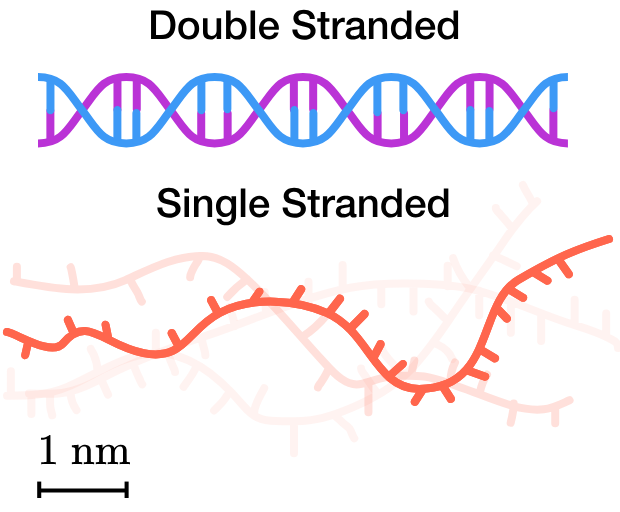A set of principles that we'll often reach for when determining biological structures is thermodynamics. The $$2^\text{nd}$$ law of thermodynamics states that a system will tend to maximize its number of configurations. Based on this thermodynamic principle, what form of DNA would you expect to be commonly found in cells?

## Exploding Genomes

### Biological Numeracy

# Exploding Genomes

Based only on maximizing the number of configurations, we'd expect single-stranded DNA to be common. But in fact the opposite is true: zoom in on a strand of DNA and it's fairly rigid. Almost all DNA is double-stranded in a cell.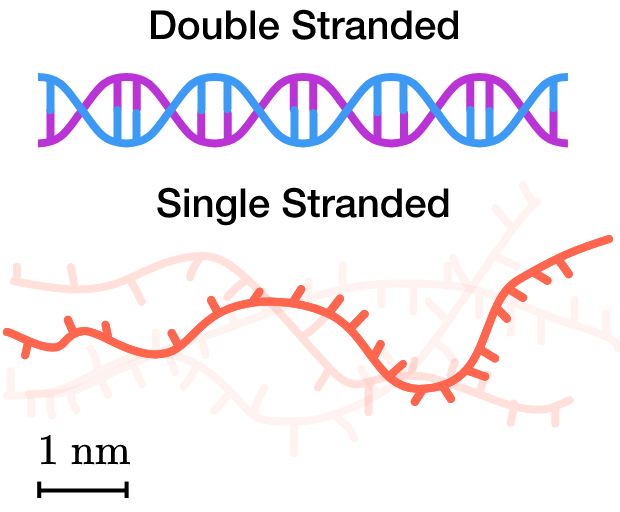Our toy model was missing something: energy. The second law of thermodynamics also says that a system will tend to minimize its energy. In biology, the relevant energy is chemical potential energy which changes when you form or break bonds. Any toy model needs to take that into account, too.

How could we model energy in our thermodynamic model to correctly predict that DNA is usually double-stranded?

## Exploding Genomes

### Biological Numeracy

# Exploding Genomes

Double-stranded DNA forms many matching bonds called base pairs between its two strands while single-stranded DNA forms no bonds at all. So even though it has fewer configurations, the bonds in double-stranded DNA pay the configuration penalty. This will be a common theme in our exploration of biological structures: most of the time, the most likely structure is the one with the most bonds.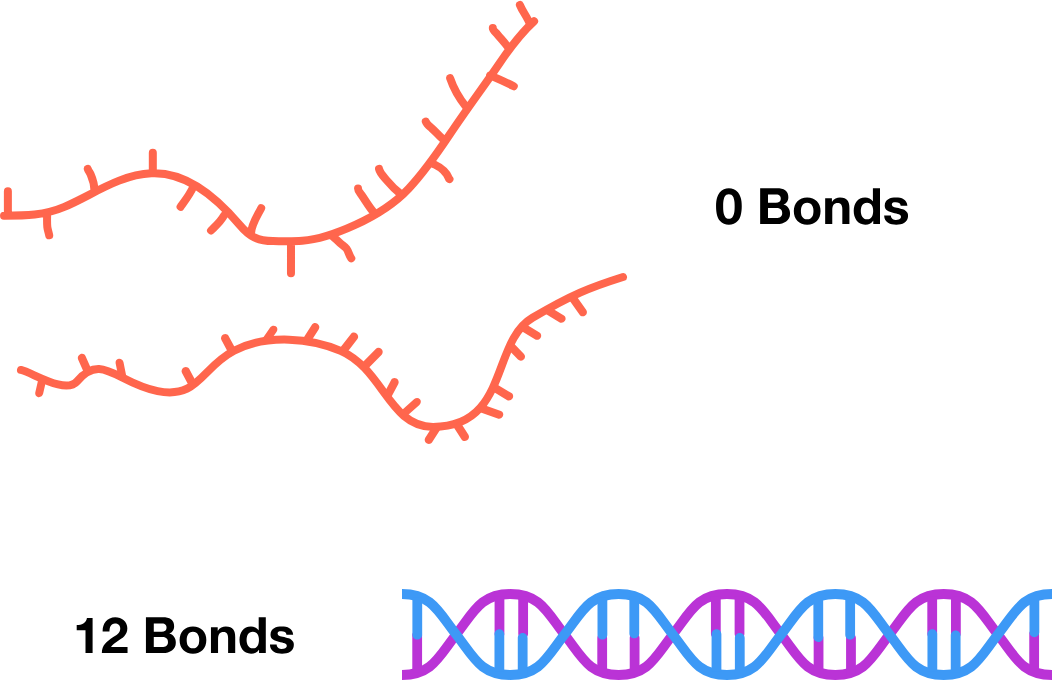Let's return to our exploded genomes. Over what distance would you estimate the rigid double-stranded DNA tends to remain straight?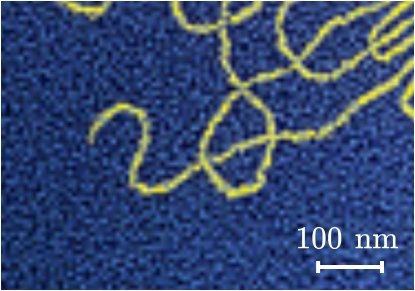## Exploding Genomes

### Biological Numeracy

# Exploding Genomes

Now that we know a bit more about the structure of DNA, we're ready to start building a toy model for the DNA blast radius left behind by exploding genomes.

We've observed that double-stranded DNA tends to stay straight over a distance of about $$\SI{50}{\nano \meter}$$. But over a longer distance, the chain can change direction. So let's model DNA as a freely jointed chain with $$n$$ links where each link $$\mathbf{r}_i$$ points in a completely random direction and has length $$b = \SI{50}{\nano \meter}$$. This toy model is often called a random walk and is a well-known problem in physics, mathematics, and finance.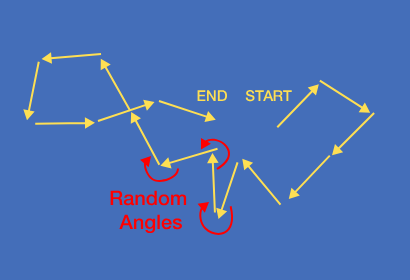When you're on a random walk, you're just as likely to go away as to come back, so on average the displacement of a long chain is zero: $\braket{\mathbf{r}} = 0.$ But still, a longer chain is more likely to spread out than a shorter one. This is measured using the mean squared displacement, the squared average spread of a random walk's path with $$n$$ links: $\braket{\mathbf{r^2}} = nb^2.$ How will the average spread of a random DNA chain depend on the $$N_{\textrm{nuc}}$$, the number of nucleotides in the chain?

## Exploding Genomes

### Biological Numeracy

# Exploding Genomes

The photos show that the length scale of the exploded human genome is about 10 times that of the bacterial genome, which in turn is about 5 times larger than the viral genome. In other words, we have $$\sqrt{N_\text{human}} \sim 10\sqrt{N_\text{bacteria}} \sim 50 \sqrt{N_\text{virus}},$$ which shows that the relative lengths are related by $$\ell_\text{human} \sim 100 \ell_\text{bacteria} \sim 2500 \ell_\text{virus}.$$

These figures are almost exactly correct.Our model can become a bit more complicated and estimate the average spread of a DNA strand in space (called the radius of gyration) in terms of its link length $$b = \SI{50}{\nano \meter}$$ and the length of each nucleotide $$\ell_n = \SI{0.34}{\nano \meter}.$$ With these parameters, the random walk model yields the number of nucleotides in these genomes given only their random spread: $\braket{r_g^2} = \frac{N_{\textrm{nuc}} \ell_n b}{6}.$ $\begin{array}{c|c|c} \ \textbf{Organism} & \textbf{Genome size (N)} \\ \hline \ \text{bacteriophage } \lambda & 149 000 \\ \ \text{Bacteria } \textit{E. coli} & 4 600 000 \\ \ \text{Human Chromosome } \textit{H. sapiens} & 120 000 000 \\ \end{array}$

## Exploding Genomes

### Biological Numeracy

# Exploding Genomes

Using this model, about how many nucleotides are in this adenovirus genome?

$\braket{r_g^2} = \frac{N \ell_n b}{6}$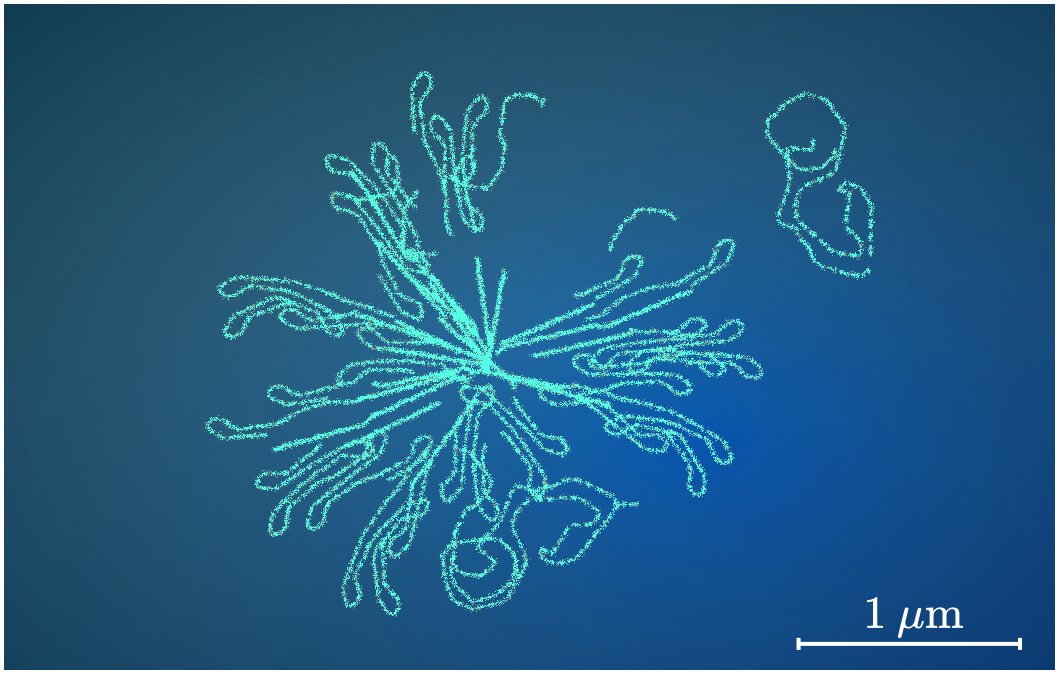## Exploding Genomes

### Biological Numeracy

# Exploding Genomes

In this course, we will develop toy models based on thermodynamics and information theory to understand and predict structures in biology. DNA is a pretty simple case—in most cases, it's a rigid rod that does little more than store information in a sequence of nucleotides.

But the hard work of biology isn't performed by DNA, but by proteins and RNA. Differences in the flexibility and chemical make-up of these molecules lead to an enormous array of different folded structures. This variety makes it much more difficult to predict the correct structure, so we'll develop more robust computational models in the Folding chapter.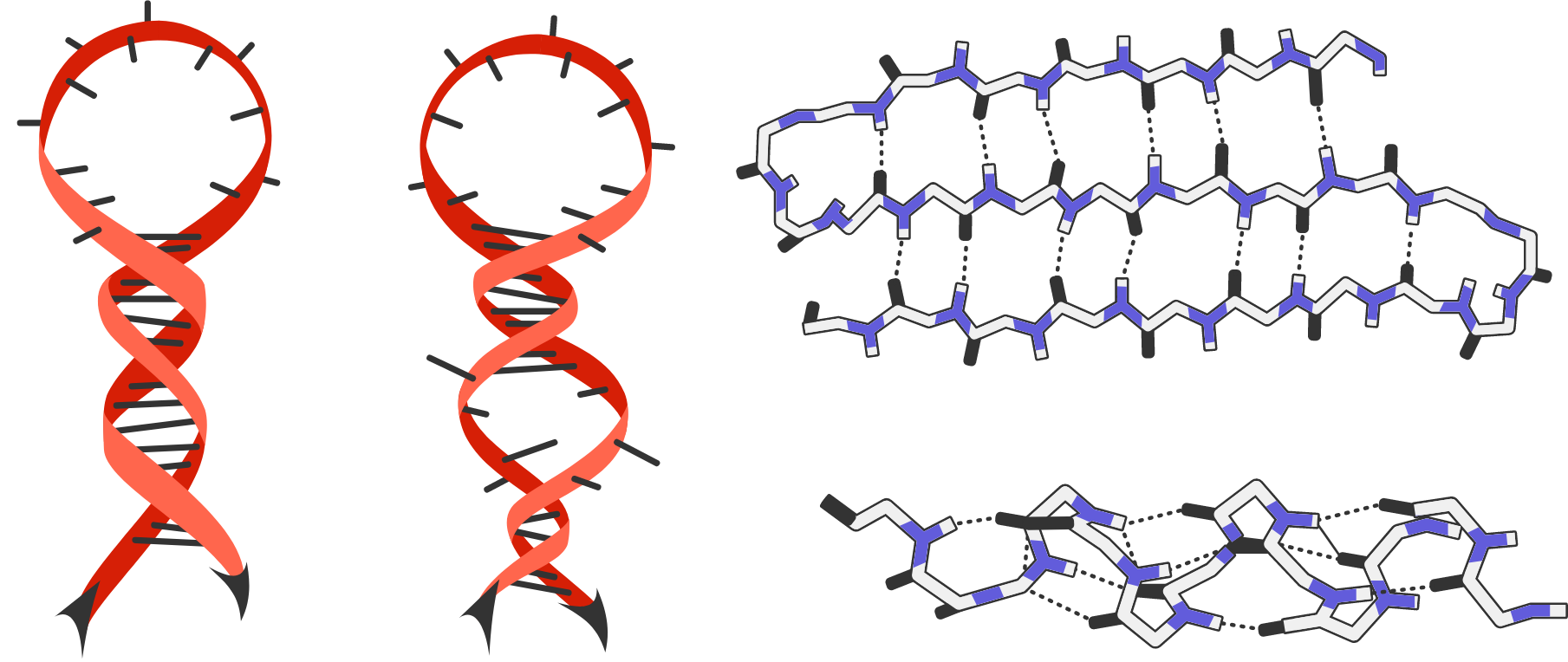## Exploding Genomes

### Biological Numeracy

×